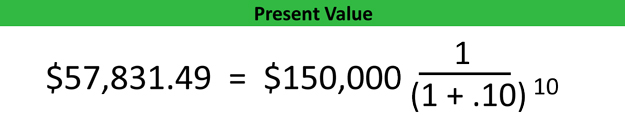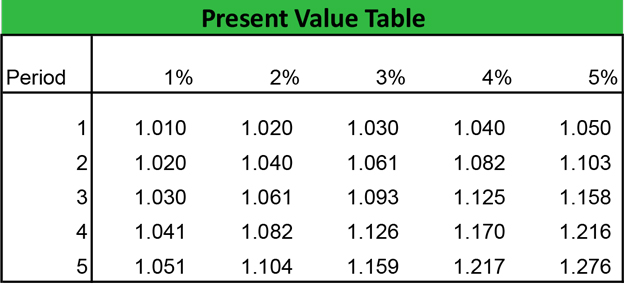## What is Present Value?

Definition? Present value, often called the discounted value, is a financial formula that calculates how much a given amount of money received on a future date is worth in today’s dollars. In other words, it computes the amount of money that must be invested today to equal the payment or amount of cash received on a future date.

This concept is based on the time value of money principle that dictates one dollar today is always worth more than one dollar tomorrow. Today’s money is always worth more than tomorrow’s because of three main reasons: interest, inflation, and opportunity costs.

The cost of cash is not free. Debtors have to pay an interest rate to creditors in order to borrow funds. Likewise, creditors’ funds are never idle. They are always earning money in the form of interest making cash a costly commodity.

Moreover, inflation devalues the purchasing power of today’s currency as time goes on. For example, a five-dollar bill in the 1950s would not be able to purchase as much in the 2020s as it could in the 1950s. Unless the five dollars is earning interest at the rate of inflation, it will slowly become worthless over time. For instance, five dollars in 1950 is actually worth about \$50 in 2015. Said a different way, a 1950 dollar is worth about 10 times a 2015 dollar. Present money is always worth more than future money.

Let’s take a look at how to calculate present value.

The present value formula is calculated by dividing the cash flow of one period by one plus the rate of return to the nth power. It sounds confusing, but it’s quite simple.Here’s what each symbol means:

• C1 = Cash flow from 1 period
• r = Rate of return
• n = Number of periods

As you can see from the present value equation, a few different variables need to be estimated. The cash flow from one period is simply the amount of money that is received on a future date. This is also called the future value of a lump sum. The rate of return is the estimated annual interest rate that will be received in the future. The number of periods is simply the number of times the interest will compound over time.

Notice that this equation uses annual interest. This means both the rate and the number of periods are in years. If you want to calculated semi-annual interest, you’ll need to divide these numbers in half.

The PV formula is often reformatted to reference the future value of the lump sum payment received like this:Here’s what each symbol means:

• FV = Future value of cash received at a later date
• r = Rate of return
• n = Number of periods

## Analysis

Both investors and creditors use a present value calculator to evaluate potential investments and measure the return on current projects. The time value of money concept is important because it allows investors to measure what their investment returns are worth today and whether there are better options available.

For instance, when someone purchases a home, they are often offered the opportunity to pay points on the mortgage to reduce insurance payments. Is it worth it? Keen investors can compare the amount paid for points and the discounted future interest payments to find out.

Another example is a lottery winner. Most lotteries will allow winners two optional payment forms. They can receive a smaller lump sum today or they can receive the full amount of winnings in equal payments for the rest of their lives. This theory is based on the time value of money. A small lump sum today is worth the larger lump sum in the future.

Company management also use this theory when investing in projects, expansions, or purchasing new equipment. By using the net present value formula, management can estimate whether a potential project is worth pursuing and whether the company will make money on the deal.

Let’s take a look at an example.

## Example

Assume Tim’s Machining is expanding its machine shop with new equipment and is in the market for a loan. Tim’s management needs a \$100,000 loan to purchase the equipment and can secure a zero interest, zero principle loan with a single \$150,000 balloon payment. How much interest is Tim actually paying?

Let’s calculate how much interest Tim will actually be paying with the balloon loan. The loan is a ten-year note, so we need to figure out what the present value of a \$150,000 lump sum is ten years from now.As you can see, the PV of the balloon payment is \$57,831.49. This means if Tim invested \$57k at 10 percent interest today, he would have enough to pay off this loan when it’s due. It also means he is paying about \$93,000 in interest.

This example assumes a single payment in the future. What about continuous annuity payments in the future?

## PV of an Annuity

Let’s go back to our lottery example. Assume that Alexa won \$1,000,000 in the state lottery. After verifying her winning ticket, the lottery commission gives her two choices for collecting her winnings. She can either collect \$426,000 now or receive \$50,000 a year for the next 20 years. What is the better deal assuming a 10 percent interest rate?

When we compute the present value of annuity formula, they are both actually the same based on the time value of money. Even though Alexa will actually receive a total of \$1,000,000 (\$50,000 x 20) with the payment option, the interest rate discounts these payments over time to their true present value of approximately \$426,000.

This is why most lottery winners tend to choose a lump sum payment rather than the annual payments.

## Present Value Tables

Typically, people use a PV calculator to compute these numbers, but they can also use a present value table. These charts compute the discount rates used in the PV calculation, so you don’t have to use a complicated equation.

All you have to do is line up interest rate listed on the x-axis with the number of periods listed on the y-axis and multiple by the payment. Here’s an example.## Say hello

#### Find us at the office

Chappa- Adamitis street no. 38, 81811 Tripoli, Libya

#### Give us a ring

Alda Runion
+69 213 130 910
Mon - Fri, 10:00-22:00